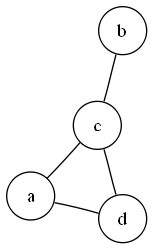# Graph Theory

In this set of articles we explore the class of objects called graphs. In its most basic incarnation a graph is a collection of vertices V and a collection of edges E. An edge is a pair of vertices (u,v) and represents a connections between those vertices. For instance, the graph given by V={a, b, c, d} and E={(a, c), (a, d), (b, c), (c, d)} is shown.Graphs are useful tools for capturing relationships between objects. In this aspect they are frequently called networks, and if this brings the internet to mind it is no coincidence. The internet can be thought of a graph whose vertices are computers or other electronic devices and whose edges represent direct connections between these devices, wired or wireless. Similarly social relationships can be thought of as graphs where vertices are individuals and edges represent specific types of relationships.

Processes on graphs, rather random or algorithmic, provide us with a toll for studying the dynamics of such real world networks: knowing how a random particle moves in a graph might tell us something about how a file moves across a network or a book or CD passed amongst friends. Allowing for more complicated processes we can study things like the spread of trends and disease. In the articles we visit some of these processes as well as look at some structural properties of graphs. These articles are self-contained and can be sampled in any order. Their difficulty varies with the random walks and flows being the most technical.

## Glossary

Here are some terms and notation that will crop up repeatedly in the articles.

• Directed graphs are graphs whose edges have an orientation. The edge (u, v) can be thought of as an arrow pointing from u to v. In this context (u, v) is not the same as (v, u). Some of the definitions below may be modified on directed graphs, but these revisions will be covered only as necessary.
• Weighted graphs are graphs whose edges are assigned some numerical value. Directed graphs can also be weighted.
• An infinite graph is one with infinitely many vertices.
• Two vertices are neighbors if there is an edge connecting them. If u and v are neighbors we write u ~ v.
• A path is an ordered set of vertices {v1, ..., vn} such vi ~ vi+1.
• The degree of a vertex v is the number of neighbors of v. This is written as deg(v).
• A combination C(n,k) is a tool for counting. If you have n numbered balls, C(n,k) is the number of ways you can chose k of them. C(n,k) = n!/[(n-k)! k!]
• For two sets A and B, the set A - B consists of the elements in A which are not in B.
• |A| denotes the number of elements in a set A.

## Solutions

Solutions for the activities in the articles can be found here